Checkout JEE MAINS 2022 Question Paper Analysis : Checkout JEE MAINS 2022 Question Paper Analysis :

# Half Wave Rectifier

Half-wave rectifiers transform AC voltage to DC voltage. A halfwave rectifier circuit uses only one diode for the transformation. A halfwave rectifier is defined as a type of rectifier that allows only one-half cycle of an AC voltage waveform to pass while blocking the other half cycle. In this session, let us know in detail about the half-wave rectifier.

### Half Wave Rectifier Circuit

A half-wave rectifier is the simplest form of the rectifier and requires only one diode for the construction of a halfwave rectifier circuit.

A halfwave rectifier circuit consists of three main components as follows:

• A diode
• A transformer

Given below is the half-wave rectifier diagram: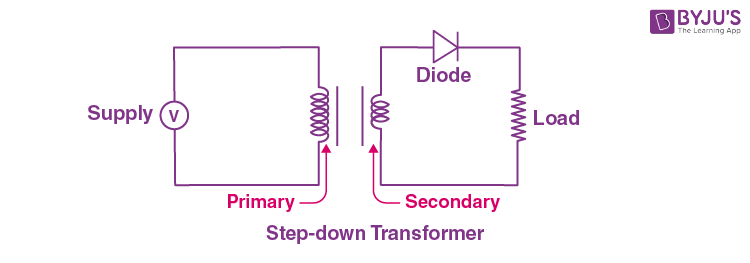### Working of Half Wave Rectifier

In this section, let us understand how a half-wave rectifier transforms AC into DC.

1. A high AC voltage is applied to the primary side of the step-down transformer. The obtained secondary low voltage is applied to the diode.
2. The diode is forward biased during the positive half cycle of the AC voltage and reverse biased during the negative half cycle.
3. The final output voltage waveform is as shown in the figure below: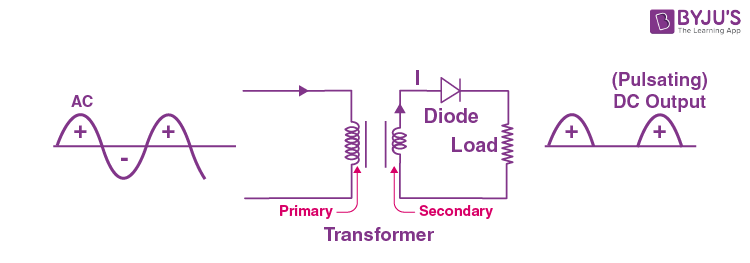For better understanding, let us simplify the half-wave circuit by replacing the secondary transformer coils with a voltage source as shown below: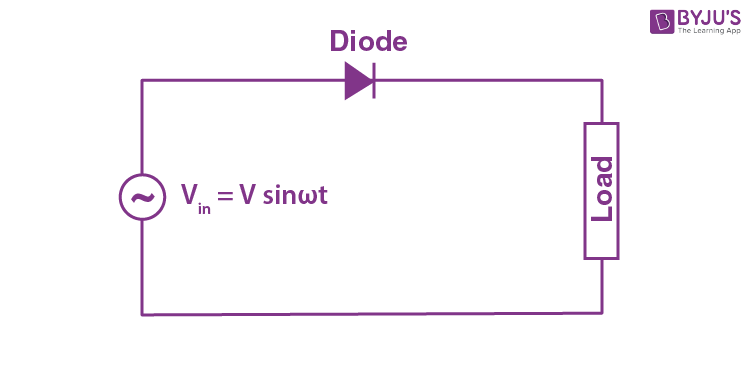For the positive half cycle of the AC source voltage, the circuit effectively becomes as shown below in the diagram: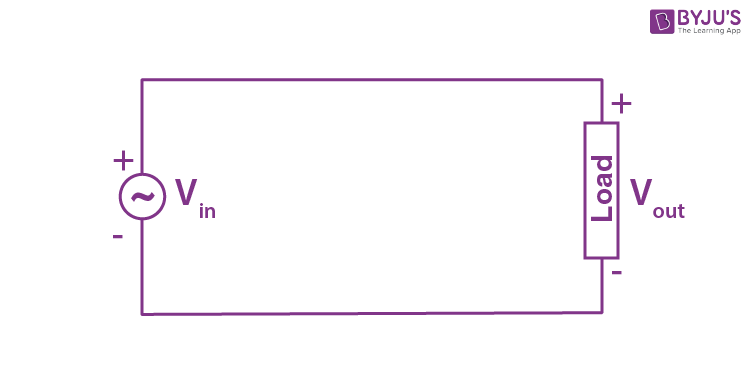When the diode is forward biased, it acts as a closed switch. But, during the negative half cycle of the AC source voltage, the equivalent circuit becomes as shown in the figure below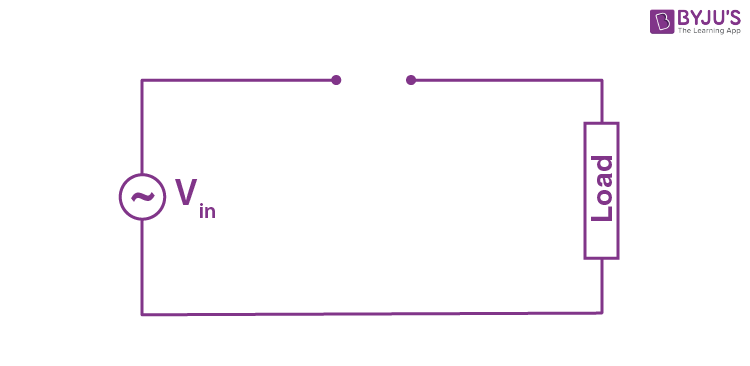When a diode is reverse biased, it acts as an open switch. Since no current can flow to the load, the output voltage is equal to zero.

### Half Wave Rectifier Waveform

The halfwave rectifier waveform before and after rectification is shown below in the figure.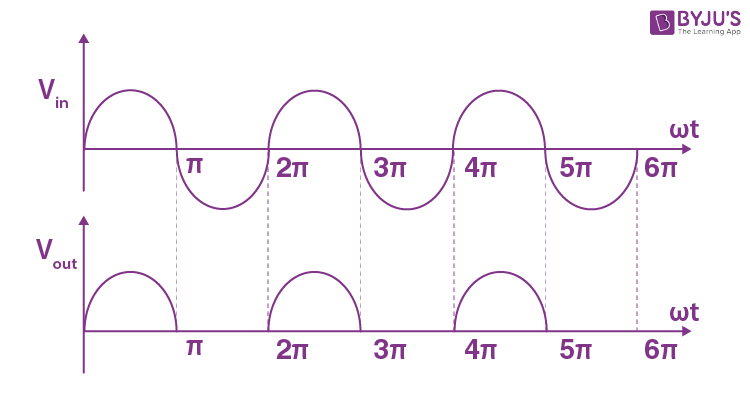## Half Wave Rectifier Capacitor Filter

The output waveform of a halfwave rectifier is a pulsating DC waveform. Filters in halfwave rectifiers are used to transform the pulsating waveform into constant DC waveforms. A capacitor or an inductor can be used as a filter.

The circuit diagram below shows how a capacitive filter is used with halfwave rectifier to smoothen out a pulsating DC waveform into a constant DC waveform.## Half Wave Rectifier Formula

### Ripple Factor of Half Wave Rectifier

Ripple factor determines how well a halfwave rectifier can convert AC voltage to DC voltage.

Ripple factor can be quantified using the following formula: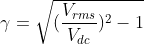The ripple factor of a halfwave rectifier is 1.21.

### Efficiency of Halfwave rectifier

The efficiency of a halfwave rectifier is the ratio of output DC power to the input AC power.

The efficiency formula for halfwave rectifier is given as follows;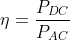### RMS value of Half Wave Rectifier

The RMS value of the load current for a half-wave rectifier is given by the formula: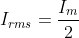### Form factor of a Halfwave Rectifier

The form factor is the ratio between RMS value and average value and is given by the formula: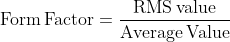## Applications of Half Wave Rectifier

Here are a few common applications of half wave rectifiers:

• They are used for signal demodulation purpose
• They are used for rectification applications
• They are used for signal peak applications

### Disadvantages of Half Wave Rectifier

• Power loss
• Low output voltage
• The output contains a lot of ripples

## Frequently Asked Questions – FAQs

### What is a halfwave rectifier?

The rectifier circuit that converts alternating current into the direct current is known as a halfwave rectifier circuit. The half-wave rectifier passes only one half of the input sine wave and rejects the other half.

### Where is a halfwave rectifier used?

A half-wave rectifier is used in firing circuits and pulse generating circuits. Halfwave rectifiers are used along with step up and step down transformers to achieve the desired voltage.

### How does a half-wave rectifier work?

Half wave rectifier circuit uses a PN junction to convert the supplied AC into DC. In a half-wave rectifier circuit, the load resistance is connected in series with the PN junction diode.

### Is the half-wave rectifier better or the full-wave rectifier?

Full-wave rectifiers are more efficient than half-wave rectifiers. A full-wave rectifier passes twice as many waves as a half-wave rectifier. Hence, more of the input is transferred to the load.

### What is the use of a filter in a half-wave rectifier?

The filter in a half-wave rectifier is used to smoothen the pulsating fluctuating DC component.

This article discussed the Halfwave rectifiers and their nuances. Stay tuned to BYJU’S and Fall in Love with Learning to learn more concepts like this!

## Why shouldn’t you touch electrical equipment with wet hands? Find out the answer to this question by watching the video.